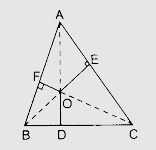# In the given figure, $O$ is a point in the interior of a triangle ABC, $OD \perp BC, OE \perp AC$ and $OF \perp AB$. Show that $AF^2 + BD^2 + CE^2 = AE^2 + CD^2 + BF^2$."

Given:

$O$ is a point in the interior of a triangle ABC, $OD \perp BC, OE \perp AC$ and $OF \perp AB$.

To do:

We have to show that $OA^2 + OB^2 + OC^2 - OD^2 - OE^2 - OF^2 = AF^2 + BD^2 + CE^2$.

Solution:

Join $OA, OB$ and $OC$In $\triangle \mathrm{AOF}$, by Pythagoras theorem,

$\mathrm{OA}^{2}=\mathrm{OF}^{2}+\mathrm{AF}^{2}$

$\mathrm{AF}^{2}=\mathrm{OA}^{2}-\mathrm{OF}^{2}$

In $\triangle \mathrm{BDO}$,

$\mathrm{OB}^{2}=\mathrm{BD}^{2}+\mathrm{OD}^{2}$

$\mathrm{BD}^{2}=\mathrm{OB}^{2}-\mathrm{OD}^{2}$

In $\triangle \mathrm{CEO}$,

$\mathrm{OC}^2=\mathrm{CE}^{2}+\mathrm{OE}^{2}$

$\mathrm{CE}^{2} =\mathrm{OC}^{2}-\mathrm{OE}^{2}$

Therefore,

$\mathrm{AF}^{2}+\mathrm{BD}^{2}+\mathrm{CE}^{2}=\mathrm{OA}^{2}-\mathrm{OB}^{2}+\mathrm{OC}^{2}-\mathrm{OD}^{2}-\mathrm{OE}^{2}+\mathrm{COF}^{2}$.......(i)

$\mathrm{OA}^{2}-\mathrm{OF}^{2}+\mathrm{OB}^{2}-\mathrm{OD}^{2}+\mathrm{OC}^{2}-\mathrm{OE}^{2}=\mathrm{AF}^{2}+\mathrm{BD}^{2}+\mathrm{CE}^{2}$

$(\mathrm{OA}^{2}-\mathrm{OE}^{2})+(\mathrm{OC}^{2}-\mathrm{OD}^{2})+(\mathrm{OB}^{2}-\mathrm{OF}^{2})=\mathrm{AE}^{2}+\mathrm{CD}^{2}+\mathrm{BF}^{2}$............(ii)
From (i) and (ii), we get,

$\mathrm{AF}^{2}+\mathrm{BD}^{2}+\mathrm{CE}^{2}=\mathrm{AE}^{2}+\mathrm{CD}^{2}+\mathrm{BF}^{2}$

Hence proved.